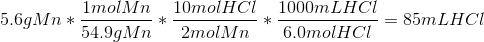# MCAT Physical : Reaction Calculations and Limiting Reagent

## Example Questions

← Previous 1 3

### Example Question #1 : Reaction Calculations And Limiting Reagent

What products will be formed by the reaction between copper (II) fluoride and sodium sulfate?

CuS and NaF

CuSO4 and NaF

Cu2Na and F2SO4

Cu2SO4 and NaF

CuSO4 and NaF

Explanation:

Recognize that this is a double replacement reaction in which ion pairs will switch. After reaction, copper (II) will now be paired with sulfate, and sodium with flouride according to the (unbalanced) equation below. Remember that sulfate has a charge of -2, and flouride of -1.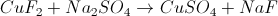### Example Question #1 : Reaction Calculations And Limiting Reagent

Which represents the correct balanced equation for the reaction between silver (I) nitrate and magnesium hydroxide?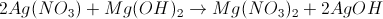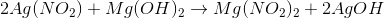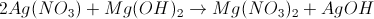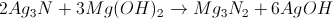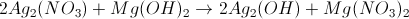Explanation:

Silver (I) nitrate is AgNO3. Recognizing this allows us to eliminate two answer choices, which incorrectly substitute nitrogen (N) for nitrate (NO3) or balance the molecular charges incorrectly. Of the two remaining choices, only one is balanced correctly.

### Example Question #3 : Reaction Calculations And Limiting Reagent

Hydrogen can be produced on a large scale by a method called the Bosch process, by which steam is passed over heated iron shavings. The reaction is shown below: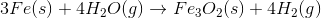Alternatively, hydrogen can be produced by reacting steam with natural gas, according to the following equation: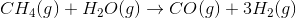How many molecules of carbon monoxide are produced per liter of hydrogen, when using the natural gas method of production at STP?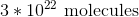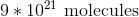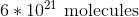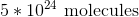Explanation:

Since the reaction takes place at STP, we can convert liters of hydrogen to moles using a constant.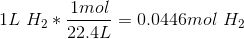Using this value, we can find the moles of carbon monoxide produced and convert to molecules by using Avogadro's number.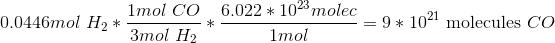### Example Question #4 : Reaction Calculations And Limiting Reagent

Hydrogen can be produced on a large scale by a method called the Bosch process, by which steam is passed over heated iron shavings. The reaction is shown below:Alternatively, hydrogen can be produced by reacting steam with natural gas, according to the following equation:Suppose equal masses of iron and methane were available, along with excess water. What is the difference in the amount of hydrogen molecules that can be produced?

Methane will produce about eight times more hydrogen molecules

Iron will produce about 1.3 times more hydrogen molecules

Both methods will produce equal amounts of hydrogen molecules

Iron will produce about eight times more hydrogen molecules

Methane will produce about eight times more hydrogen molecules

Explanation:

To determine which method will produce more hydrogen molecules, convert equal masses of each reactant into molecules of hydrogen gas. The conversion requires us to convert the initial compound to moles, multiply by the molar ratio from the reaction to find moles of hydrogen, and multiply by Avogadro's number to convert to molecules.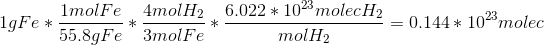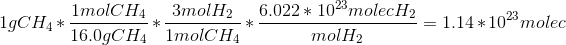Dividing these solutions, we see that the methane method produces roughly eight times the amount of hydrogen molecules.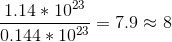### Example Question #5 : Reaction Calculations And Limiting Reagent

Hydrogen can be produced on a large scale by a method called the Bosch process, by which steam is passed over heated iron shavings. The reaction is shown below:Alternatively, hydrogen can be produced by reacting steam with natural gas, according to the following equation:Suppose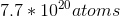of iron react with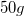of steam to create iron oxide and hydrogen. What is the limiting reagent and how much of the excess reactant remains?

Iron is the limiting reagent and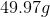of steam will remain

Iron is a catalyst, and will not be consumed

Iron is the limiting reagent and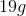of steam will remain

Steam is the limiting reagent and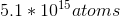of iron will remain

Iron is the limiting reagent andof steam will remain

Explanation:

In order to identify an excess reactant, we use stoichiometry to convert atoms of iron to mass of steam.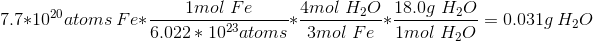We haveof steam available, but only require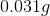to fully react the given iron. Iron is thus the limiting reagent, since it will be fully consumed first.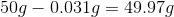There will beof excess steam.

### Example Question #1 : Reaction Calculations And Limiting Reagent

How many milliliters of 0.5M NaOH are required to react completely with 10g of BaCO3?

200mL

400mL

50mL

100mL

200mL

Explanation:

First, write a balanced equation for the reaction.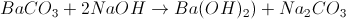Next, convert 10g BaCO3 to milliliters of NaOH.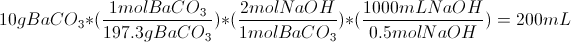### Example Question #7 : Reaction Calculations And Limiting Reagent

Which of the following produces a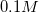solution of potassium chloride?

Put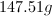of solute into a container and bring the volume to one liter by adding water while stirring

Potassium chloride is not soluble in water

Put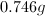of solute into a container and bring the volume to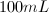by adding water while stirring

Add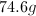of solute to one liter of water

Add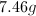of solute toof water

Putof solute into a container and bring the volume toby adding water while stirring

Explanation:

We will be looking for a solution that results in one mole of potassium chloride per ten liters of water.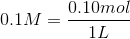We will need to find the molecular weight of potassium chloride.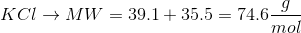In order to get the desired concentration, we will need to add one-tenth of this amount to one liter of water.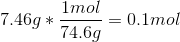Our ratio, then is: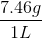The only answer to follow this ratio is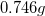of potassium chloride in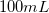.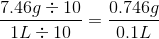### Example Question #8 : Reaction Calculations And Limiting Reagent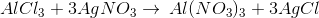If you begin with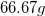of aluminum chloride and unlimited silver nitrate, how many grams of silver chloride can be produced?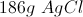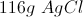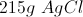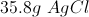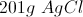Explanation:

This is a stoichiometry question requiring us to convert between grams, moles, reactants, and products.

Use the periodic table to find the molar masses of the two compounds in question.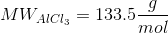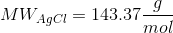We can use the reaction formula to find the ratio of aluminum chloride to silver chloride. In this case, the ratio is 1:3.Now we can set up a calculation to convert grams of aluminum chloride to grams of silver chloride, making sure that all units cancel appropriately.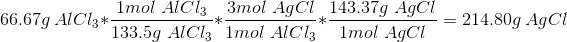### Example Question #9 : Reaction Calculations And Limiting Reagent

Hydrogen can be produced on a large scale by a method called the Bosch process, by which steam is passed over heated iron shavings. The reaction is shown below:Alternatively, hydrogen can be produced by reacting steam with natural gas, according to the following equation:Which hydrogen production method would be more efficient in areas where water is scarce?

The natural gas method is more efficient because for every one mole of water consumed, three moles of hydrogen are produced

Both methods would be equally efficient in the described environment

The iron method is more efficient because it produces four moles of hydrogen gas

The iron method is more efficient because it utilizes a common metal

The natural gas method is more efficient because for every one mole of water consumed, three moles of hydrogen are produced

Explanation:

The question asks us to consider water scarcity. Assuming our goal is to utilize minimal water to produce maximal hydrogen, the natural gas method is most efficient as is produces more hydrogen per mole of water consumed.One mole of water produces three moles of hydrogen.Four moles of water produce four moles of hydrogen.

### Example Question #10 : Reaction Calculations And Limiting Reagent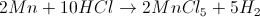5.6g of manganese reacts with 650mL of 6.0M hydrochloric acid to form manganese (V) chloride and hydrogen gas. Along with the products, a large amount of heat is evolved.

What is the limiting reagent, and how much of the excess reagent will remain after the reaction?

HCl is limiting; 0.3g excess Mn

There is no limiting reagent

HCl is limiting; 0.15g excess Mn

Mn is limiting; 370mL excess HCl

Mn is limiting; 565mL excess HCl